﻿

### On not open linear continuous operators between Banach spaces

#### Abstract

Let X and Y be infinite-dimensional Banach spaces. Let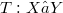$T: X → Y$ be a linear continuous operator with dense range and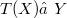$T(X) ≠ Y$. It is proved that, for each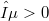$ε > 0$, there exists a quotient map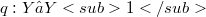$q: Y → Y1$, such that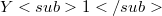$Y1$ is an infinite-dimensional Banach space with a Schauder basis and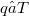$q ○ T$ is a nuclear operator of norm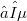$≤ ε$. Thereby, we obtain with respect to the quotient spaces the proper analogue result of Kato concerning the existence of not trivial nuclear restrictions of not open linear continuous operators between Banach spaces. As a consequence, it is derived a result of Ostrovskii concerning Banach spaces which are completions with respect to total nonnorming subspaces.

DOI Code: 10.1285/i15900932v25n1p29

Keywords: Not open linear continuous operators; Nuclear operators; Basic sequence; Biorthogonal system; Quotient spaces

Classification: 46B20; 47A65; 47B10

Full Text: PDF